# Mathematic Year 11 Ext Term 2 Exam

• Inverse functions
• Roots of an equation
• Long division

Questions.

Question 1. [1 mark]

Question 2. [3 mark]

Divide the equation 2x3 – 5 x2 – 8x + 15 by (x-3) using long division

Question 3.

Question 4.

The polynomial 4x3 + 8x2 – 14 x +8 has three roots α, β and γ

a) Find αβγ(α+β+γ) [1 marks]

b) Find α2 + β2 + γ2 [3 marks]

Question 5.

What is the remainder when x3 – 3x2 + 3 is divided by x – 4

Question 6.

Find the polynomial Q(x) that satisfies x3 + 2x2-3x -8 = (x-2)Q(x)  +2

Question 7.

Find the inverse function, y = (x2 – 4) / y

Question 8.

Question 1. C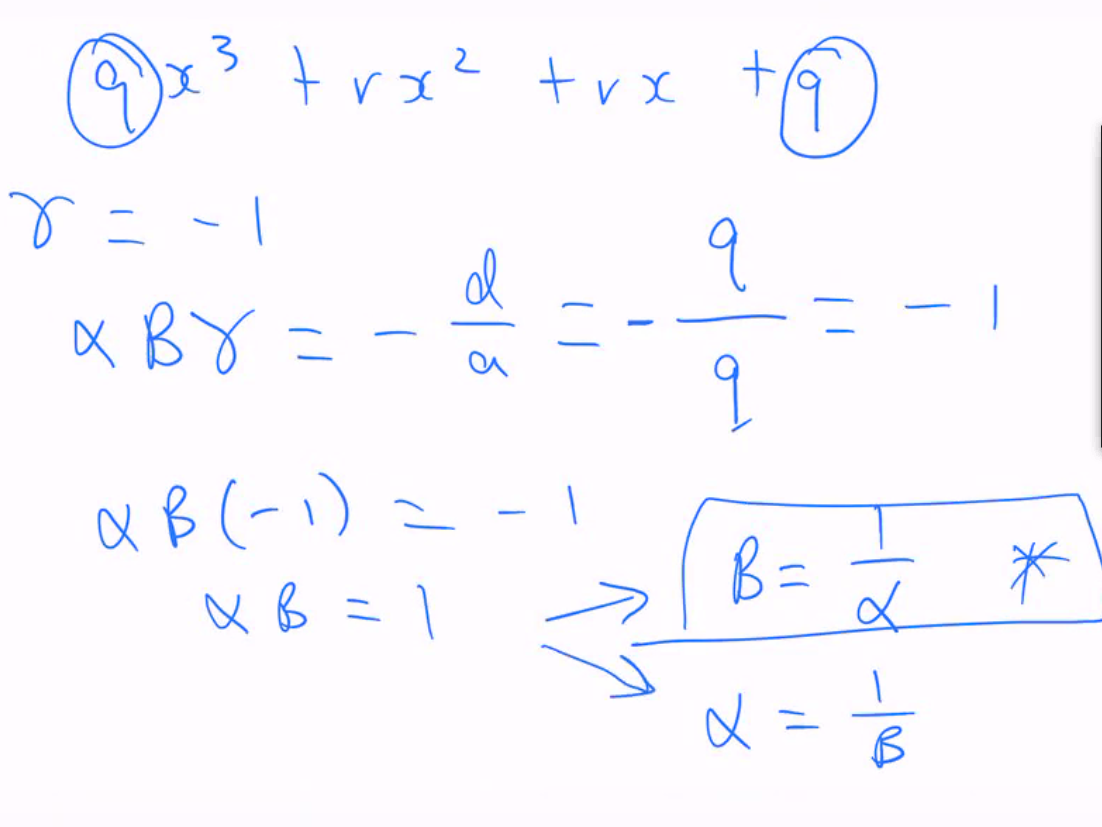Question 2.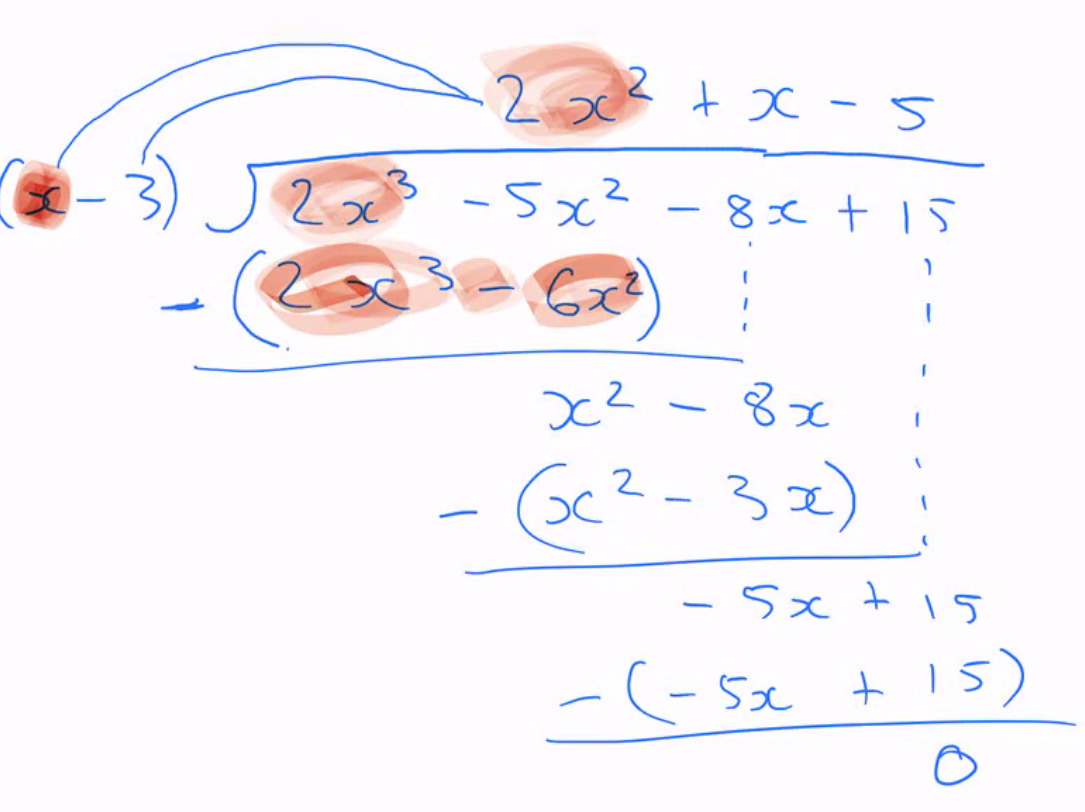Therefore, 2x2 + x -5 is the answer

Question 3.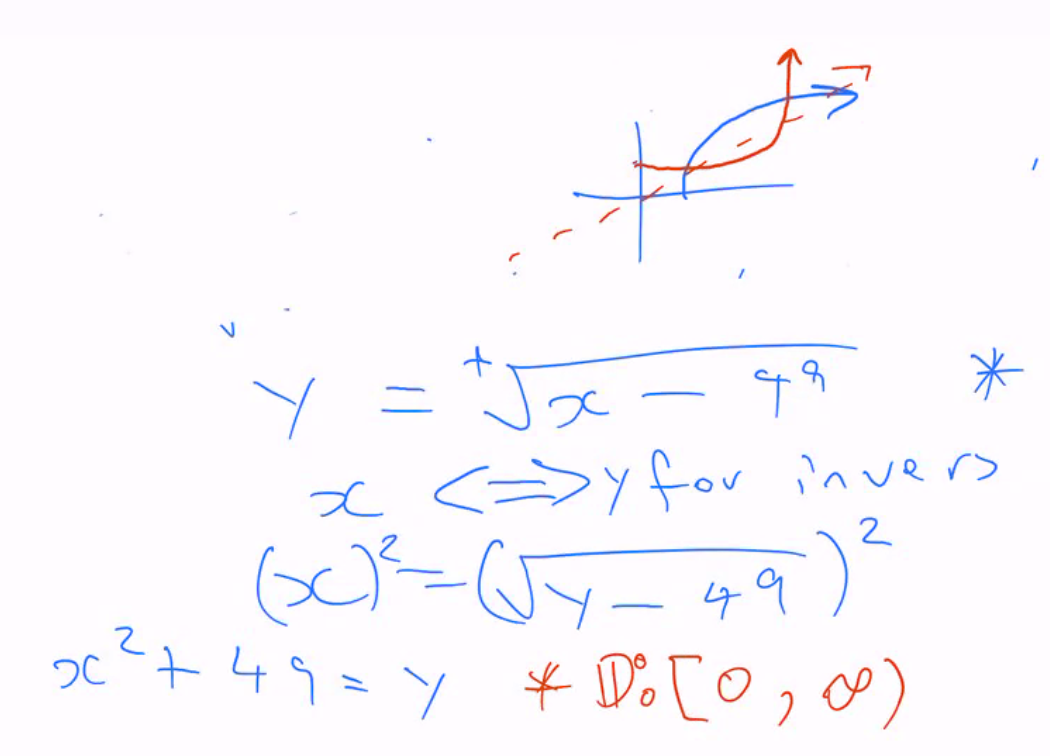Question 4.

a) 4

b)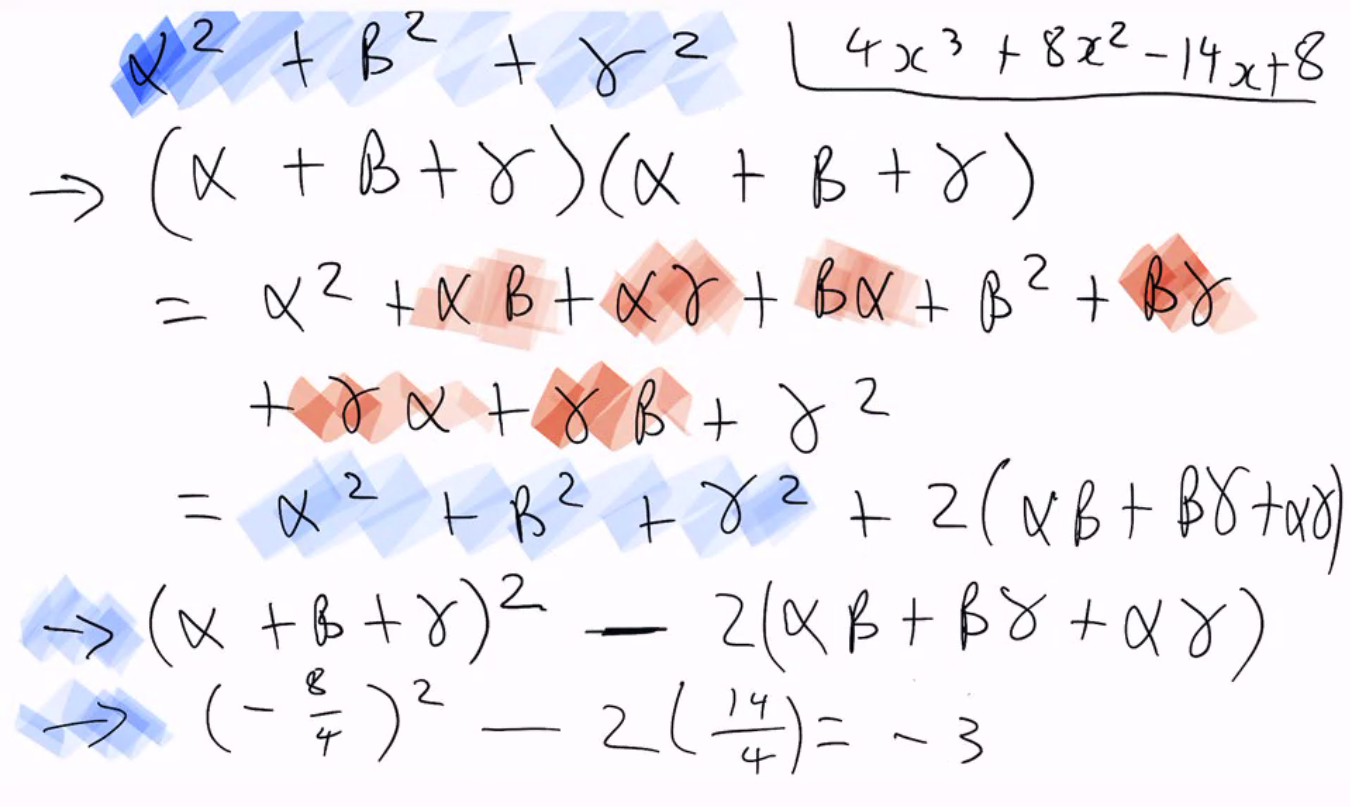Question 5.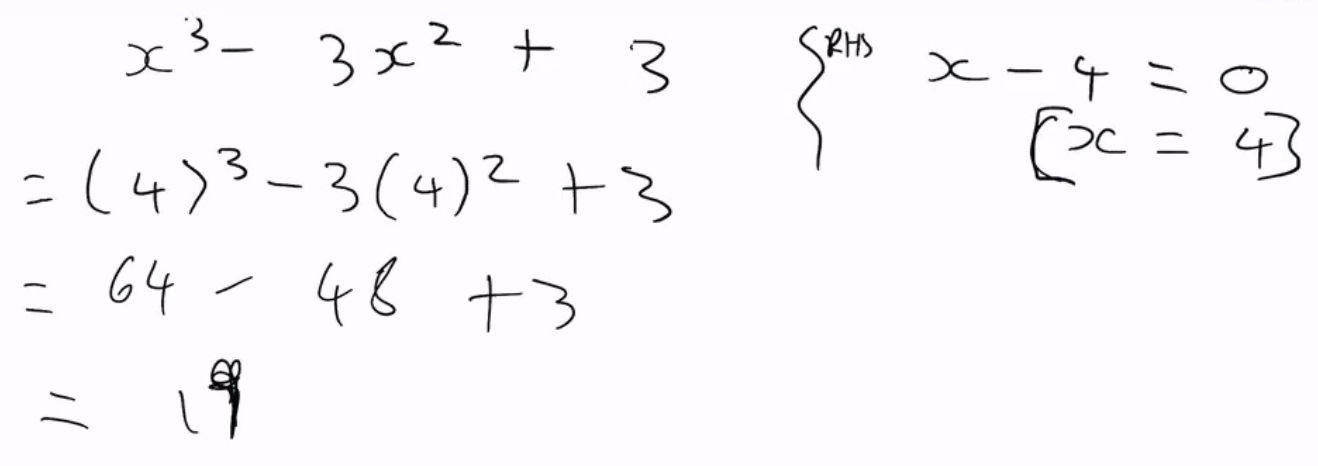Question 6.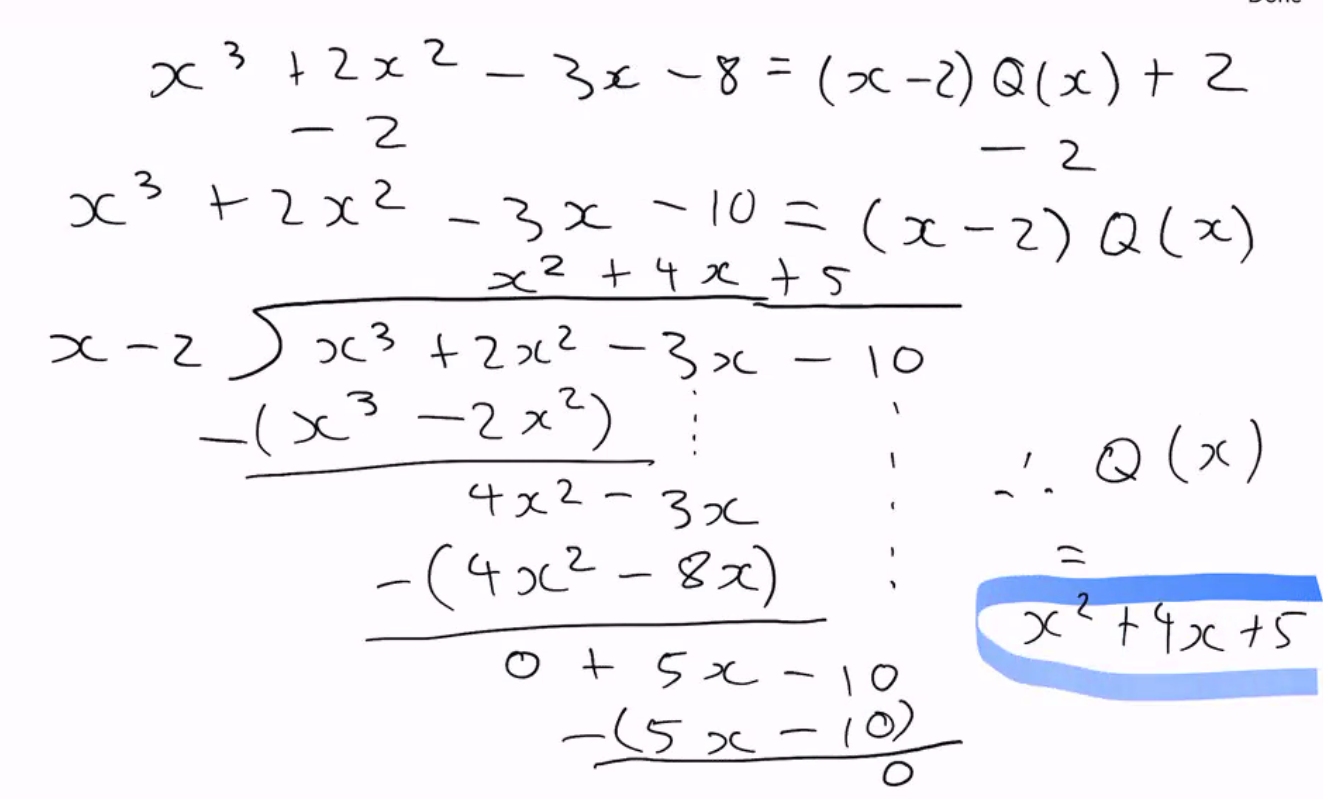Question 7.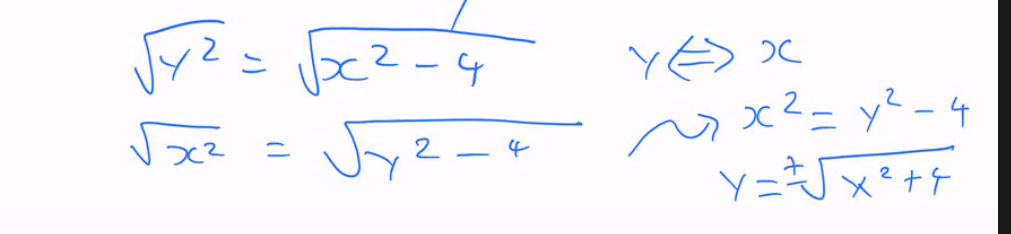Question 8.

A –> (-3)^3 -6*-3 = -9 Is the remainder